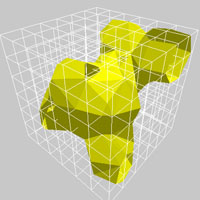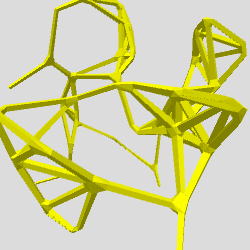# ComputationalGeometry

### 3D Geometry Library for Processing.org

Hosted by the Cloud Lab
Columbia University Graduate School of Architecture, Planning & Preservation.

This library provides Processing1 support for dynamic mesh generation and rendering, including isometric contours and surfaces, boundary hulls and skeletons. Isometric shapes are defined "implicitly" and are representative of threshold conditions within a gradient and can be realized at any level of detail. They are often used to represent heights in a topographic map, mesh reconstructions from MRI scans, proteins surface models and pressure lines in weather maps. This implementation works in a meta-ball modeling framework but also alows you to override the underlying data structure.
1 Processing.org is a framework for algorithmic visualization and computational sketching. It was created at the MIT Media Lab by Ben Fry and Casey Reas.

## Installation

Unzip and put the extracted ComputationalGeometry folder into the libraries folder of your Processing sketches. Reference and examples are included in the ComputationalGeometry folder.

Keywords. 3D, geometry, surface, contour, iso, polygon, poly, generative, Proxy

Reference. Have a look at the javadoc reference here. A copy of the reference is included in the .zip as well.

Source. The source code of ComputationalGeometry is available at GitHub, and its repository can be browsed here.

## Getting Started

There are a few stages to getting a mesh surface to show up on the screen. First you need to init the surface, with some surfaces needing bounds information and detail settings. Next, seed the surface by adding points or edges to it. Lastly, generate the mesh by supplying the plot function with any needed values such as thresholds or thicknesses.

An example IsoSurface:

```import ComputationalGeometry.*;

//Constructing the IsoSurface
IsoSurface iso = new IsoSurface(this, new PVector(0,0,0), new PVector(100,100,100), 8);

for(int i=0; i<10; i++){
PVector pt = new PVector( random(100), random(100), random(100) );

// Alternate: add a point with a weighting factor
}

// Plotting
iso.plot(mouseX/10000.0);```
An example IsoContour:

```import ComputationalGeometry.*;

//Construct the Isocontour
IsoContour iso = new IsoContour(this, new PVector(0,0), new PVector(100,100), 10,10);

// Adding Data to the Isocontour
randomSeed(1);
for(int i=0; i<10; i++){
PVector pt = new PVector( random(100), random(100), 0 );

// Alternate: add a point with a weighting factor
}

// Plot Isocontour
fill(255,0,0);
iso.plot( max(mouseX/800.0, 0.05) );```
An example IsoSkeleton:

```import ComputationalGeometry.*;

//Constructing the IsoSkeleton
IsoSkeleton skeleton = new IsoSkeleton(this);

// Create points to make the network
PVector[] pts = new PVector;
for (int i=0; i<pts.length; i++) {
pts[i] = new PVector(random(-100, 100), random(-100, 100), random(-100, 100) );
}

for (int i=0; i<pts.length; i++) {
for (int j=i+1; j<pts.length; j++) {
if (pts[i].dist( pts[j] ) < 60) {
}
}
}

// Plotting
skeleton.plot(5.0f, .25f);  // Thickness as parameter```
An example IsoWrap:

```import ComputationalGeometry.*;

// Constructing the IsoWrap
IsoWrap wrap = new IsoWrap(this);

// Create points to be wrapped
PVector[] pts = new PVector;
for (int i=0; i<pts.length; i++) {
pts[i] = new PVector(random(-100, 100), random(-100, 100), random(-100, 100) );
}

// Plotting
wrap.plot();
```

## Examples

Find a list of examples in the current distribution of ComputationalGeometry, or have a look at them by following the links below.

## Tested

Platform osx
Processing 3.0### Contributors

@ProxyMark Mark Collins is co-director of Proxy, an innovation-focused design firm working across a range of scales and platforms. Mark is as an adjunct assistant professor at the Columbia University Graduate School of Architecture where he co-directs the Cloud Lab.

@proxyToru Toru Hasegawa is co-director of Proxy. Toru investigates the culture of innovation and technology in architecture as an adjunct assistant professor at the Columbia University Graduate School of Architecture Toru is a co-director of the Cloud Lab.

Please direct all inquiries to inquiry @ thecloudlab dot org